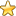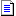The Main Number Sections:
0 - 500 | 501 - 1000 | 1001 - 1500 | 1501 - 2000 | 2001 - 2500
Number Articles | Higher Numbers | Random Number
You are here: HomeThe Database of Number Correlations# Partition Theory

Partition theory is a fundamental area of number theory. It is concerned with the number of ways that a whole number can be partitioned into whole number parts.

5 for example can be partitioned in 7 ways thus:
11111, 2111, 221, 311, 32, 41, 5.
The permutations of these 7 partitions add up to 16 thus: 11111 =1 permutation 2111 =4 permutations 221 =3 permutations 311 =3 permutations 32 =2 permutations 41 =2 permutations 5 =1 permutation

A partition is a way of writing an integer n as a sum of positive integers where the order of the parts is not significant. Number Partitions Order counts 0 111112223344585716611327156482212893025610425121156 1024 1277 2048 13101 4096 14135 8192 15176 16384 16231 - 17297 - 18385 - 19490 - 20627 - 21792 - 22 1002 -
http://home.att.net/~numericana/data/partition.htm Partition Numbers for 0 to 4096 (site down?)

# 5, 7, 11 Congruences: Properties and Meanings

Starting with 4, the number of partitions for every 5th integer is a multiple of 5-eg the number of partitions for 9 is 30 and for 14 is 135.

Starting with 5, every 7th integer is a multiple of 7.

Starting with 6, every 11th integer is a multiple of 11.

# Other Relationships: Properties and Meanings

Number of partitions of n into an even number of distinct parts equals the number of partitions of n into an odd number of distinct parts-except when n is a Pentagonal Number.(Franklin?)

Number of partitions of n into parts not divisible by 3>than the number of partitions of n into parts none of which occurs more than twice.

The number of odd partitions of n=the number of distinct partitions of n.

The total number of 1s that occur among all unordered partitions of a positive integer is equal to the sum of the numbers of distinct members of those partitions.

SEQUENCE: 0, 1, 3, 6, 12, 20, 35, 54, 86, 128, 192, 275, 399, 556, 780, 1068, 1463, 1965, 2644, 3498, 4630, 6052, 7899, 10206, 13174, 16851, 21522, 27294, 34545, 43453, 54563, 68135, 84927, 105366, 130462, 160876, 198014, 242812, 297201, 362587, 441546, 536104, 649791, 785437, 947812, 1140945, 1371173, 1644136, 1968379, 2351597, 2805218, 3339869, 3970648, 4712040, 5584141, 6606438, 7805507, 9207637 ...
The total number of parts in all partitions of n. Also sum of all large parts of all partitions of n.

SEQUENCE: 0, 0, 1, 3, 7, 13, 24, 39, 64, 98, 150, 219, 322, 455, 645, 892, 1232, 1668, 2259, 3008, 4003, 5260, 6897, 8951, 11599, 14893, 19086, 24284, 30827, 38888, 48959, 61293, 76578, 95223, 118152, 145993, 180037, 221175, 271186, 331402, 404208, 491521...
The number of "+" signs needed to write the partitions of n.

Number of partitions of n into distinct parts >= 2....number of partitions of n into odd parts.
1, 1, 1, 2, 2, 3, 4, 5, 6, 8, 10, 12, 15, 18, 22, 27, 32, 38, 46, 54, 64, 76, 89, 104, 122, 142, 165, 192, 222, 256, 296, 340, 390, 448, 512, 585, 668, 760, 864, 982, 1113, 1260, 1426, 1610, 1816, 2048, 2304, 2590, 2910, 3264, 3658, 4097, 4582, 5120, 5718
http://www.research.att.com/~njas/sequences/?q=distinct+parts&sort=0&fmt=0&language=english&go=Search

You are here: HomeThe Database of Number CorrelationsThe Main Number Sections:
0 - 500 | 501 - 1000 | 1001 - 1500 | 1501 - 2000 | 2001 - 2500
Number Articles | Higher Numbers | Random Number